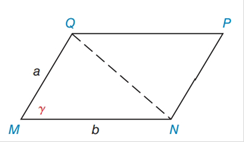Chapter 11.4, Problem 40E### Elementary Geometry for College St...

6th Edition
Daniel C. Alexander + 1 other
ISBN: 9781285195698

#### Solutions

Chapter
Section### Elementary Geometry for College St...

6th Edition
Daniel C. Alexander + 1 other
ISBN: 9781285195698
Textbook Problem
1 views

# Find the area of ▱ M N P Q  if  a = 8  cm,  b = 12  cm, and  γ = 70 ∘ . Answer to the nearest tenth of a square centimeter. (See Exercise 39.)To determine

To find:

The area of the MNPQ

If a=8cm, b=12cm, and γ=70

Explanation

1) Formula:

The area of the parallelogram is given by,

A=absinγ unit2.

Where, a is the measure of a sides, and b is the base of the triangle,

γ is the angle between the base and the side.

2) Calculation:

Given,

The area of the MNPQ is given by,

A=absinγ unit2

Here, a=8cm, b=12cm, and γ=70

A=(8)(12

### Still sussing out bartleby?

Check out a sample textbook solution.

See a sample solution

#### The Solution to Your Study Problems

Bartleby provides explanations to thousands of textbook problems written by our experts, many with advanced degrees!

Get Started

#### Subtract the following numbers. 17.

Contemporary Mathematics for Business & Consumers

#### Which curve is simple but not closed?

Study Guide for Stewart's Multivariable Calculus, 8th

#### The area of the region bounded by , , and r = sec θ is:

Study Guide for Stewart's Single Variable Calculus: Early Transcendentals, 8th